• Introduction
• What is in this manual
• What is Caspoc
• User interface
• Introduction
• Starting
• Simulation
• Editing
• Viewing and printing
• Getting Started
• Basic editing
• Simulation in the time domain
• Basic User Interface Topics
• Editing
• Simulation
• Viewing
• Library
• Reports
• Project management
• Circuit and Block Diagram Components
• Introduction
• Cscript and user defined functions
• Component parameters
• Modeling Topics
• Introduction
• Power Electronics
• Semiconductors
• Electrical Machines
• Electrical drives
• Power Systems
• Mechanical Systems
• Thermal Systems
• Magnetic Circuits
• Green Energy
• Coupling to FEM
• Experimenter
• Analog hardware description language
• Embedded C code Export
• Coupling to Spice
• Small Signal Analysis
• Matlab coupling
• Tips and tricks
• Appendices

## Fuel Cell based on a voltage-current lookup table characteristic

Modeling a Fuel Cell based on the Voltage/Current Density graph.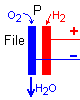The model is based on the Voltage/Current Density characteristic provided by the manufacturer of the Fuel Cell.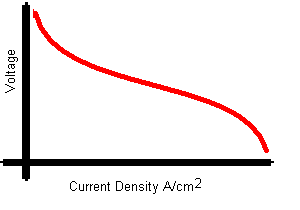The manufacturer provides this data per stack. This data is used in Caspoc and forms the basis of the Fuel Cell model.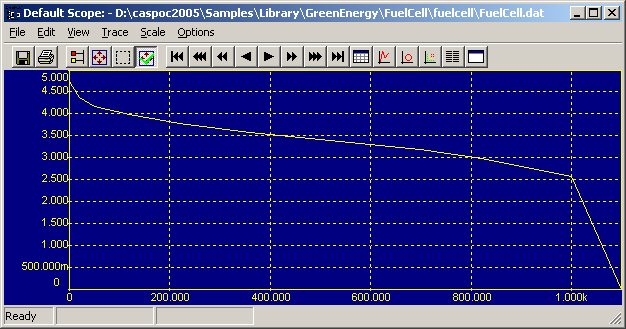On the X-axis the current density in mA/cm2. The y-axis is the voltage per stack. By defining the number of stacks and the area of the stack, the total power produced by the fuel cell can be calculated. The voltage of the fuel cell is depending on the current drawn form the fuel cell as indicated by the graph above.

The Fuel Cell library block is selected from the library at Components/Library/GreenEnergy/FuelCell

In the sample section is an example of a fuel cell connected to a boost converter.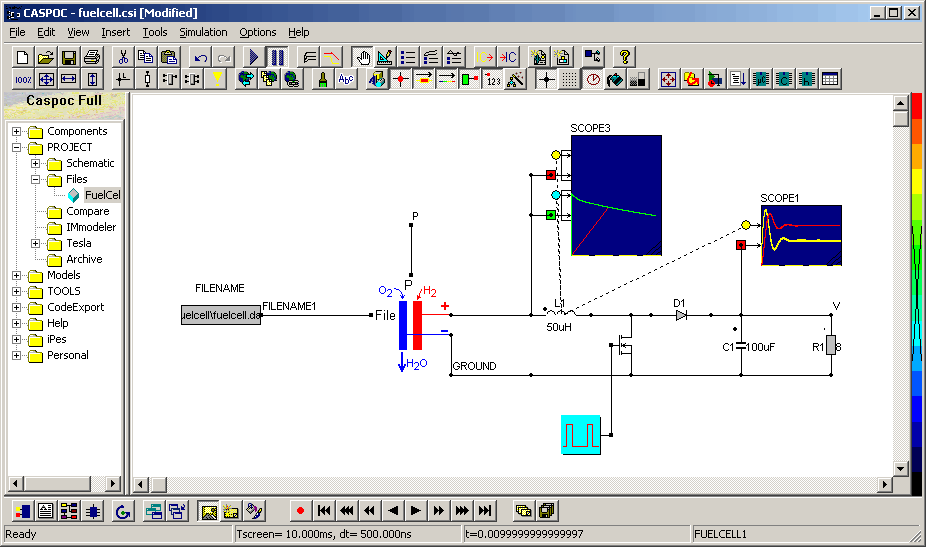It has 4 terminals. On the left side is the terminal for the name of the data file describing the characteristic. It should be connected to a block called FILENAME that is selected from Components/Blocks/Miscellaneous. In the block Filename you have to specify the file name for the characteristic at the input field Text2: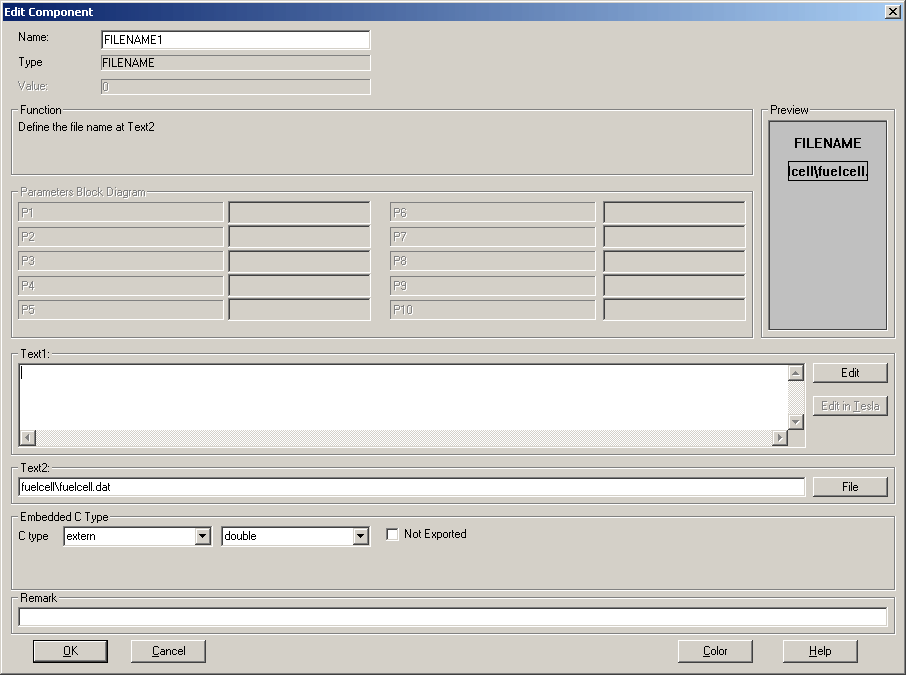On the right side are the electrical terminals that are connected to the boost converter. The connection on the top of the fuel cell is for displaying the amount of power that is delivered by the fuel cell. This signal can be displayed in a scope or used as a signal in a block diagram.

The Fuel Cell block has two parameters, the active area per cell and the number of stacks.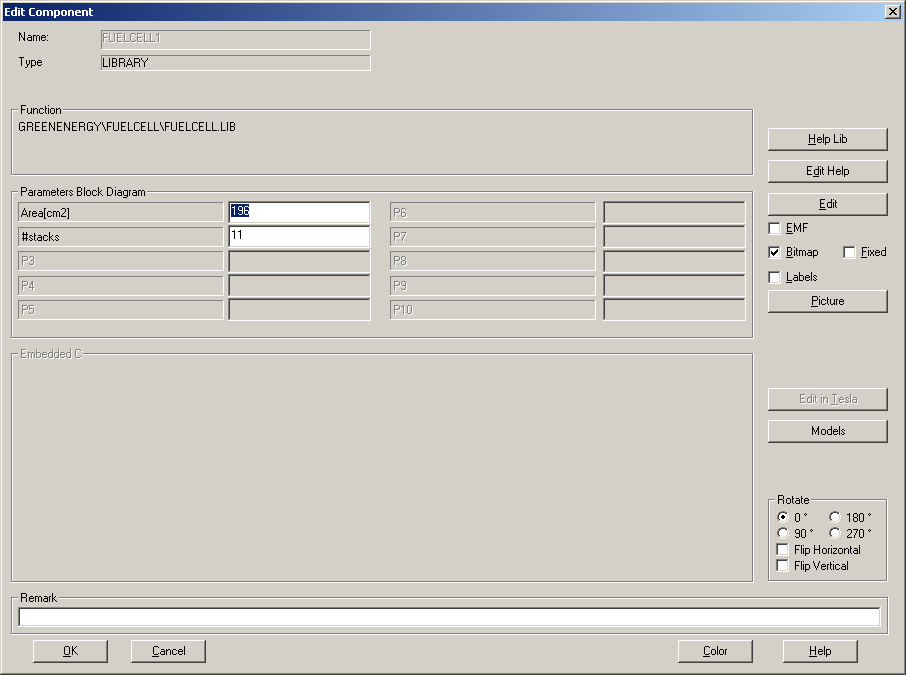The active area is given in cm2 and the number of stacks is given by the parameter #stacks.

• Active Area
• The active area is smaller than the total size of the fuel cell. The current drawn from the fuel cell equals the product of the current density and the active area. In our example the steady state is around 25 Ampere. This means that the current density in the fuel cell equals 25/196=128mA/cm2.
• Number of stacks
• The number of stacks is internally divided by 5, because in many cases the characteristic is given for 5 stacks. Therefore the number of stacks has to be multiplied by n if the characteristic is given for n stacks.
• Internal resistance
• The internal resistance of the fuel cell is fixed and set to 25mOhm. The internal voltage loss equals 25m*25=0.625V From the characteristic we see that for a current density of 128mA/cm2 the open circuit voltage for 11 stacks will approximate 11/5 * 4=8.8volts. Taking the voltage drop of 0.625volt over the internal resistance into account, the terminal voltage approximates 8.2 volts.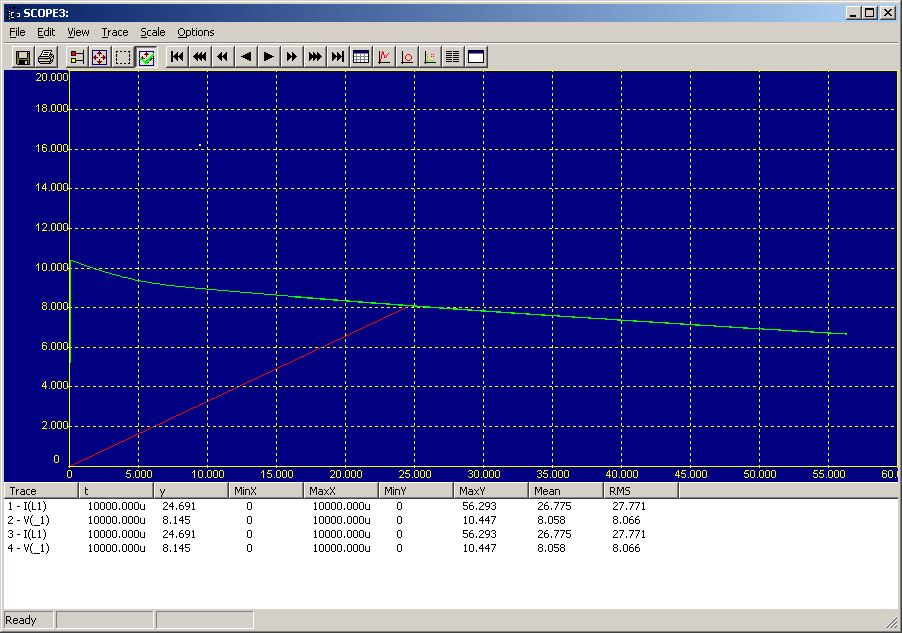The amount of power delivered by the fuel cell equals 8.1Volt*25Ampere=204 Watt. This value can be read from the terminal on top of the fuel cell.